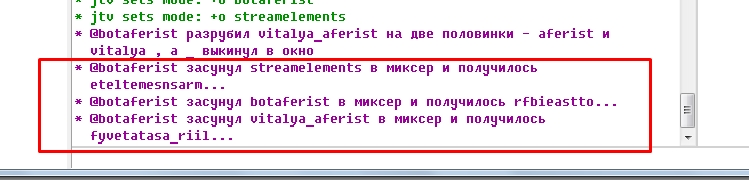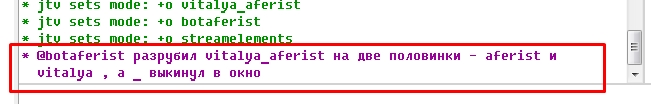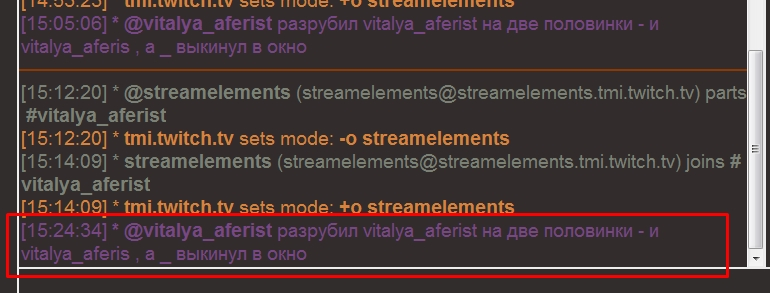## [Help] problems with mirc script

I know that there are problems with \$calc but here I have two scripts, and in one everything is good, and in another problem, I do not know why
in the first script everything is fine (do not look at Russian words), he mixes the letters in the wordmirc``````mixer {
var %t = \$1, %was
while (%t != \$null) {
var %x = \$rand(1,\$len(%t))
var %was = %was \$+ \$mid(%t,%x,1)
%t = \$left(%t,\$calc(%x - 1)) \$+ \$right(%t,\$calc(\$len(%t) - %x))
}
return %was
}

``````

and the second in mirc normally works, but in adiirc problemsmirc

here the script cuts the nickname ))
and if in the nickname there is _ then the script divides its right side puts in the beginning, and the left one to the right and _ throws it out the window))but here the right part is not taken at all, and the left one takes all up to the penultimate character, but at the same time _ is discarded normally

``````popolam {
; если длина ника равна 1 букве
if (\$len(\$1) == 1) { return me расчленил \$\$1 на много половинок }
; если длина больше 2 и точка содержится при делении длины на 2, т.е. мы проверяем четное слово или нет
if (\$len(\$1) > 2) && (\$chr(46) isin \$calc(\$len(\$\$1) / 2)) { return me разрубил \$\$1 на две половинки - \$right(\$\$1,\$calc(\$len(\$\$1) / 2)) и \$left(\$\$1,\$calc(\$len(\$\$1) / 2)) , a \$mid(\$1,\$int(\$calc((\$len(\$1) /2)+1)),1) выкинул в окно }
; если число четное
else { return me разрубил \$\$1 на две половинки - \$right(\$\$1,\$calc(\$len(\$\$1) / 2)) и \$left(\$\$1,\$calc(\$len(\$\$1) / 2)) }
}

``````

what can be the problem and how to fix
Thanks!

### Replies (2)

#### RE: [Help] problems with mirc script - Added by Per Amundsenabout 5 years ago

The problem is actually not \$calc.

In mIRC, floating point numbers such as 1.5 in \$mid/\$left/\$right seems to be converted to int, this is what's causing the problems.

I will fix this for the next beta, for now you can change \$calc(\$len(\$\$1) / 2)) to \$int(\$calc(\$len(\$\$1) / 2))) which removes the decimals.

#### RE: [Help] problems with mirc script - Added by greeple greepleabout 5 years ago

actually, I also thought about \$mid/\$left/\$right, but decided to ask what and how because in the wiki by the identifiers and in adi and in mirc one and the same
Thanks!

(1-2/2)